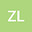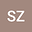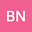The approximate analytical solution of the point source problem in 2D heterogeneous media
•••• +1Shengsheng Zhang
University of Science and Technology of China
Author ProfileBenoît Noetinger
University of Science and Technology of China
Author ProfileXiaohong Wang
University of Science and Technology of China
Author Profile## Abstract

The approximate analytical solution of the point source problem in 2D heterogeneous media is provided in this article. It is based on the analytical solution of 2D quasi-Laplace equation, which can be expressed as a combination of piecewise power series. Each power series is expanded at a singular point with a group of intrinsic non-integer power exponents, and then they are sewn together to obtain the solution for the whole region. To permit the power series to be used in the region containing point sources, the point source is approximately treated as a closed inner boundary, on which Neumann boundary condition is imposed. By this means, the approximate analytical solution of the point source problem, which is actually the Green's function for 2D Poisson equation, is obtained.